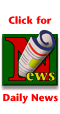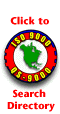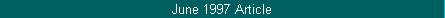by Donald J. WheelerFirst, you have to detect a difference before you can meaningfully estimate that difference, and only then can you assess the practical importance of that difference.Capability ratios are statistics. All statistics will vary from sample to sample, even when the underlying process doesn't change. We will use the data in Table 1 to illustrate the manner in which capability ratios vary over time. The data in Table 1 were obtained using a quincunx (also known as a bead board). Since no changes were made in the settings of the bead board while these 500 data were collected, these data should be taken as being typical of data from a process that displays a reasonable degree of statistical control. And that is exactly what we see in Figure 1a chart that reveals a predictable process.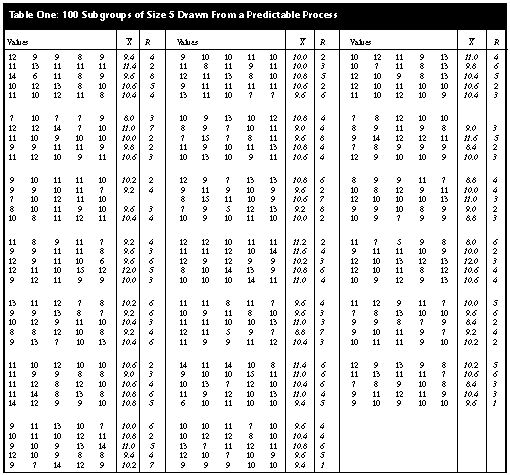For the data of Table 1, the grand average is 10.084, and the average range is 4.11. Thus, the limits for an average and range chart for these data would be:For subgroup averages:X= ± A2 R-  =  10.084  ±  (0.577)(4.11) = 7.71 to 12.46For subgroup ranges:D4 R-  =  (2.114)(4.11)  =  8.69As may be seen in Figure 1, the data of Table 1 do, indeed, display a reasonable degree of statistical control. There are no indications of anything other than routine, common-cause variation.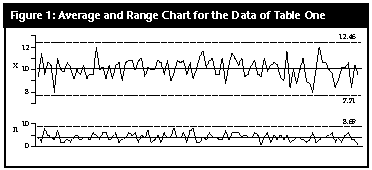Based on these data, the natural process limits would be computed as:X=  ± 3R-/d2 =  10.084  ±  3 (1.767)  =  4.78 to 15.39meaning that we should expect process values that range from 5 to 15. If the specification limits for this process are 3 to 17, then we will have a process in the ideal state -- both predictable and conforming. With these specification limits and the summary statistics from the control chart, the capability ratio for this bead board process would be:Cp  =  (USL-LSL)/6Sigma(X)  =  (17-3)/(6.0)(1.767)  =  1.32With a grand average of 10.084, the centered capability ratio is:Cpk   =  (USL-X=)/3Sigma(X)  =  (17-10.084)/(3.0)(1.767)  =  1.30Today, many customers ask for centered capability ratios of 1.33 or greater. While this process is close, it does not quite meet this magic value.The calculations above are based on 500 data, and we seldom wait until we have so much data to compute the capability ratios. In most cases, capabilities are computed occasionally, or periodically, using 50 or fewer values. So we shall divide our 100 subgroups into blocks of 10 subgroups each and compute the Cpk value for each block. Ten subgroups of size 5 will yield estimates of dispersion with about 36 degrees of freedom, which is commonly considered to be satisfactory.  Subgroups 1 to 10: Grand average = 10.08,average range = 4.0, and Sigma(X) = 4.0/2.326  =  1.720The centered capability ratio is:   Cpk =  (17.0-10.08)/(3.0)(1.720)  =  1.34A good capability ratio -- our customer will be pleased with this. Subgroups 11 to 20: Grand average = 9.98, average range = 3.9, and Sigma(X) = 3.9/2.326  =  1.677The centered capability ratio is:    Cpk =  (9.98-3.0)/(3.0)(1.677)  =  1.39Congratulations, the capability ratio got better. Subgroups 21 to 30: Grand average = 10.12, average range = 4.5, and Sigma(X) = 4.5/2.326  =  1.935The centered capability ratio is:    Cpk =  (17.0-10.12)/(3.0)(1.935)  =  1.19What happened to cause the capability ratio to drop? Subgroups 31 to 40: Grand average = 10.24, average range = 4.2, and Sigma(X) = 4.2/2.326  =  1.806The centered capability ratio is:    Cpk =  (17.0-10.24)/(3.0)(1.806)  =  1.25This capability ratio is still not good enough -- we must do better. Subgroups 41 to 50: Grand average = 10.10, average range = 4.9, and Sigma(X) = 4.9/2.326  =  2.107The centered capability ratio is:    Cpk =  (17.0-10.10)/(3.0)(2.107)  =  1.09This is worse than before, and the third value is below 1.33 -- things had better improve or else! Subgroups 51 to 60: Grand average = 10.42, average range = 3.9, and Sigma(X) = 3.9/2.326  =  1.677The centered capability ratio is:    Cpk =  (17.0-10.42)/(3.0)(1.677)  =  1.31Well, this is better, but our customer is asking for 1.33 or greater. Subgroups 61 to 70: Grand average = 10.30, average range = 4.7, and Sigma(X) = 4.7/2.326  =  2.021The centered capability ratio is:    Cpk =  (17.0-10.30)/(3.0)(2.021)  =  1.11You were supposed to be improving -- what is going on here? Subgroups 71 to 80: Grand average = 10.06, average range = 3.8, and Sigma(X) = 3.8/2.326  =  1.634The centered capability ratio is:    Cpk =  (17.0-10.06)/(3.0)(1.634)  =  1.42At last -- send these data to our customer. Subgroups 81 to 90: Grand average = 9.88, average range = 3.5, and Sigma(X) = 3.5/2.326  =  1.505The centered capability ratio is:    Cpk =  (9.88-3.0)/(3.0)(1.505)  =  1.52Excellent capability ratio. Good work. Subgroups 91 to 100: Grand average = 9.66, average range = 3.7, and Sigma(X) = 3.7/2.326  =  1.591The centered capability ratio is:    Cpk =  (9.66-3.0)/(3.0)(1.591)  =  1.40Why is this value slipping?    Of course, the real answer is that we are not slipping. The process was not getting worse, nor was it getting better. But our statistics were changing from block to block even though the process remained the same, and these changes were reflected in the centered capability ratio values computed. If we placed these 10 centered capability ratios on an XmR chart, we would get Figure 2.Figure 2 shows that the routine amount of variation in capability ratios, based upon 50 values each, will allow them to go as low as 0.88 or as high as 1.72, while the process is unchanging with an average capability ratio of 1.30.     Before you can interpret a single capability ratio, based upon 50 data, to mean that this process capability has changed from the value of 1.30, the single ratio would have to fall below 0.88 or it would have to go above 1.72! First, you must detect a difference before you can meaningfully estimate that difference, and only then can you assess the practical importance of that difference. To ignore this sequence is to risk interpreting noise as if it were a signal, with the result that your interpretation will turn out to be meaningless and your actions inappropriate. So how can you make sense of the capability ratios? Put them on a control chart. If this chart is out of control, you can be sure that your process is also out of control. However, the converse is not true -- your process can be out of control and still yield a control chart for capability ratios that is in control.    To allow for the inherent variation in any statistic is the beginning of numerical literacy. To fail to do so is the primary symptom of numerical illiteracy. You will reveal the group to which you belong by the way you interpret capability ratios.About the author     Donald J. Wheeler is an internationally known consulting statistician and the author of Understanding Variation: The Key to Managing Chaos and Understanding Statistical Process Control, Second Edition. He can be reached by e-mail at dwheeler@qualitydigest.com.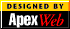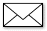Copyright 1997 QCI International. All rights reserved. Quality Digest can be reached by phone at (916) 893-4095.Please contact our Webmaster with questions or comments.[Homepage]